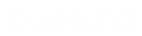Feedback

• Contents

# Monitored Values

Director uses post-call and pre-call monitored values to make routing decisions.

## Post-call monitored values

The following table describes the post-call monitored values.

 Monitored Value Description Interaction Priority Interaction priority weight (-10 to 10 inclusive).The default value is 1. Lower numbers mean less important, negative numbers mean that a higher number lowers the score. Interaction Time in Queue Interaction time in queue weight (-10 to 10 inclusive, default 1). Lower numbers mean less important, negative numbers mean that a higher number lowers the score. Interaction Time in System Interaction time in system weight (-10 to 10 inclusive, default 1). Lower numbers mean less important, negative numbers mean that a higher number lowers the score. Agent Skills Agent skills weight, which is the overall weight assigned to the overall skills score, not the weight per skill. The range is -10 to 10 inclusive. The default is 1. Lower numbers mean less important, negative numbers mean that a higher number lowers the score. Agent Cost Agent cost weight (-10 to 10 inclusive, default -1). Lower numbers mean less important, negative numbers mean that a higher number lowers the score. Agent Available Time Agent available time weight (-10 to 10 inclusive, default 1). Lower numbers mean less important, negative numbers mean that a higher number lowers the score. Agent Occupied Percentage Amount of time that an agent is busy with ACD calls and After Call Work, relative to the amount of ACD logon time for that day. The formula is: ACD Talk + ACW / ACD Logged In, where ACD Talk is total time that the agent is on ACD calls (that day), added to the total time that the agent is in ACW status (that day), divided by the total amount of time that the agent is logged on and available to accept ACD calls (that day). Ratio of Free to Active Lines Ratio of free lines to active lines weight (-10 to 10 inclusive, default 1). Lower numbers mean less important, negative numbers mean that a higher number lowers the score. Queue Member Order Weight Queue member order weight (-10 to 10 inclusive, default 0). Lower numbers mean less important, negative numbers mean that a higher number lowers the score). Routing Cost Routing cost weight (-10 to 10 inclusive, default -1). Lower numbers mean less important, negative numbers mean that a higher number lowers the score. Specific Agent Scoring factor weight for a specific Agent. The default bias is positive. The default weight is 1. Specific Workgroup Scoring factor weight for a specific Workgroup. The default bias is positive. The default weight is 1. Specific Server Scoring factor weight for a specific Server. The default bias is positive. The default weight is 1.

## Pre-call monitored values

The following table describes the pre-call monitored values.

 Monitored Value Description Number of Logged in Agents Number of logged-on agents weight (-10 to 10 inclusive, default 1). When the bias is positive, lower numbers mean less important. When the bias is negative, a higher number lowers the score. Longest Wait Longest amount of time a call waited for an available agent to pick up. Average Wait Average amount of time a call waited for an available agent to pick up. Number of Waiting Calls Number of waiting calls weight (-10 to 10 inclusive, default -1). Lower numbers mean less important, negative numbers mean that a higher number lowers the score. Number of Connected Calls Number of connected calls weight (-10 to 10 inclusive, default -1). Lower numbers mean less important, negative numbers mean that a higher number lowers the score. Ratio of Free to Active Lines Ratio of free lines to active lines weight (-10 to 10 inclusive, default 1). Lower numbers mean less important, negative numbers mean that a higher number lowers the score. Number of Active Lines Number of active lines weight (-10 to 10 inclusive, default -1). Lower numbers mean less important, negative numbers mean that a higher number lowers the score. Number of Wrapup Agents Number of wrap-up agents weight (-10 to 10 inclusive, default 0). Lower numbers mean less important, negative numbers mean that a higher number lowers the score. Average Wrapup Time Average wrap-up time weight (-10 to 10 inclusive, default 0). Lower numbers mean less important, negative numbers mean that a higher number lowers the score. Agent Skills Logged-on agent skills weight, which is the overall weight assigned to the overall skills score, not the weight per skill. The range is -10 to 10 inclusive. The default is 1. Lower numbers mean less important, negative numbers mean that a higher number lowers the score. Queue Member Order Weight Queue member order weight (-10 to 10 inclusive, default 0). Lower numbers mean less important, negative numbers mean that a higher number lowers the score.